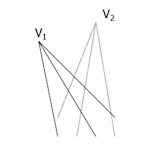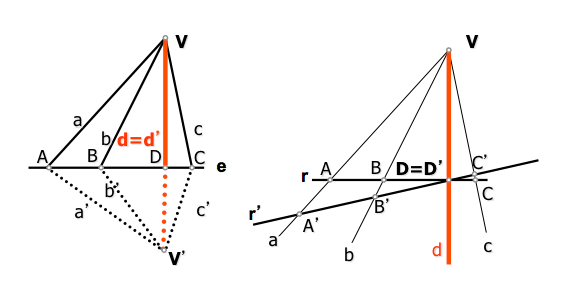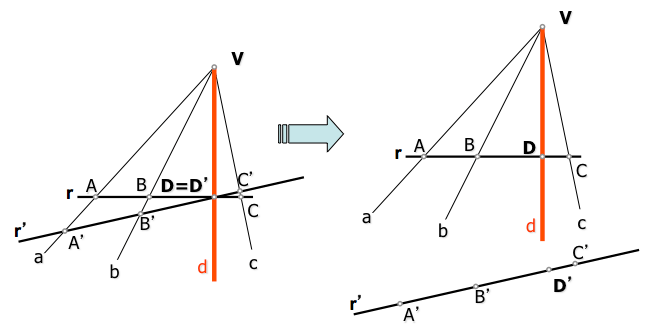# Geometría proyectiva: ProjectivityThe relationship called cuaterna” or “double ratio of four elements” to define the general homographic transformations perspectivity and projectivity.

We have seen, to study the perspectivity between forms of first category, that a number of base v and haz vertex V, not located on the line v, are perspectival if the series is section of the beam or, which is the same, if the beam is projected from the vertex V of the base series v.

This notion of perspectivity between elements notch, but of different nature (points, straight), we have defined for similar items (beam lines and series of points), generalizing the concept of perspectivity subsequently geometric elements of the same type:Two straight beams different vertices, V.. and V..‘, perspective are each other, as may be obtained as a projection of a common set.

Two series of points different bases, s and s‘, prospects are each other, as may be obtained as a single beam section.

In both cases we see that the geometric forms and related, series o haces, have a common dual element (the point straight Doubles).

• Straight beams V..(abcd…) and In '(a’b’c’d’…), de bases V.. and In ', are perspectival perspectival axis with the straight and. The common line to V and V ', that contains the bundles bases, es un dual element: d=d’
• The series of points r(ABCD…) and r’(A’B’C’D’…), de bases r and r’ , are perspectival perspectival center point with V. The common point between r and r ', containing a series of bases, es un dual element: D=D’

### Projective Methods

By moving two bundles perspectival status perspectivity is lost, however, to not change the relative position between the elements of each form, quaternions remain:

(abcx)=(ABCX)=(a’b’c’x’)We say that the bundles of vertices V and V’ quaternions are projective if four elements that determine one and the other beam counterparts are equal (have the same characteristic).

In the case of two perspectives series have the same result. If we separate by moving two series are the same beam section, cease to outlook but remain equal quaternions, being therefore each projective.In this case, if we form a quad with four points of the series and one with his counterparts from the other series will be fulfilled:

(ABCD) = (A´B´C´D´)

We will see later how we can operate with this series and beams by perspectividades intermediate, getting what we will call “projective centers and axes